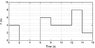# Reading a Force vs Time graph to get distance?

• ceziacrowe
In summary: So the equation is x = (1/2)*a*t^2 +Vi*t + Xi, where Vi is the final velocity of the previous calculation. Keep doing this for each section and adding up the total displacement for all sections. In summary, the net force exerted on a 3.5-kg block is shown in a graph as a function of time. Using the equations of F=ma and x = (1/2)*a*t^2 +Vi*t + Xi, the block travels a total distance of 10.838 m in the first 11.0 seconds. It is important to change the initial velocity each time when calculating displacement for different sections of the graph.

## Homework Statement

The net force exerted on a 3.5-kg block is shown in the figure as a function of time. How far does the block travel in the first 11.0 s? Assume that the motion is one dimensional and that the velocity of the block at time t= 0 s is 0 m/s.

F=ma

## The Attempt at a Solution

I figured that I could use the force on the graph so I used F=ma. Using the first point of the graph, I got 4 N = (3.5 kg)a, solved for a and got 1.14 m/s^2. Since I'm trying to find distance, I tried using Vf^2 = Vi^2 + 2ad but here's where I got stuck. Initial velocity would be 0 m/s but for final velocity, I'm not sure where I would get that number from. Am I headed in the right direction with this problem?

Also, this type of graph is kind of confusing to read for me. If the y-axis in this problem was labeled acceleration (ax) instead of F(N), a straight horizontal line from 0 to 2 seconds would be constant acceleration then from 2 to 6 seconds, it would be zero acceleration?

Any help would be amazing and immensely appreciated.

#### Attachments

•prob01av10.gif
10.8 KB · Views: 998
Last edited:
Use this equation: x = (1/2)*a*t^2 +Vi*t + Xi

And, a horizontal line is constant acceleration, A line horizontal at Zero is zero acceleration.

Texag said:
Use this equation: x = (1/2)*a*t^2 +Vi*t + Xi

And, a horizontal line is constant acceleration, A line horizontal at Zero is zero acceleration.

So I calculated each part and it's still coming out as an incorrect answer.

For 0-2 s, x=(1/2)(1.14 m/s^2)(2^2 s) + 0 m/s + 0.
I did the same thing (finding a then plugging it into that equation) until the 11.0 s mark on the graph but the answer was incorrect.

I also put for Xi the total amount of X calculated so far but I got the same answer of 10.838 m.

Last edited:
You have to be sure to change the initial velocity each time you calculate. For example, when finding the displacement of the second portion, It is not zero, but the final velocity of the one you calculated above.